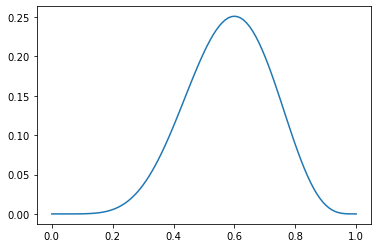# 概率与似然

## 概率

P(5次正面朝上)=C^5_{10}0.5^5(1-0.5)^5=0.24609375\approx0.25

# 最大似然估计

P = C^6_{10}0.5^6(1-0.5)^4=0.205078125\approx0.21

P=C^6_{10}0.6^6(1-0.6)^4=0.25082265600000003\approx0.25